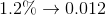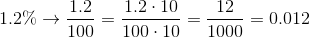# Algebra 1 : How to find decimal equivalent to a percentage

## Example Questions

### Example Question #31 : How To Find Decimal Equivalent To A Percentage

Which of the following is the decimal equivalent of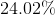?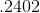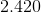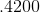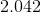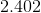Explanation:

To find the decimal equivalent of a percentage, simply move the decimal (if there is none, remember that there is always an implied decimal after the one's digit) to the left twice.  The best answer is:### Example Question #32 : How To Find Decimal Equivalent To A Percentage

Which of the following is the decimal equivalent of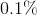?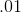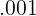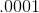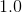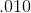Explanation:

To find the decimal equivalent of a percentage, simply move the decimal (if there is none, remember that there is always an implied decimal after the one's digit) to the left twice.  The best answer is:### Example Question #33 : How To Find Decimal Equivalent To A Percentage

If I still owe my friend Ralphof thedollars he loaned me, how much money do I still owe Ralph?  Round your answer to the nearest cent.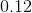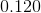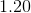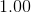Explanation:

To solve this problem, first, you must convert the percentage above into a decimal.  To find the decimal equivalent of a percentage, simply move the decimal (if there is none, remember that there is always an implied decimal after the one's digit) to the left twice.  Then, you take your result and multiply it by the initial amount for your answer:### Example Question #34 : How To Find Decimal Equivalent To A Percentage

If you owe the bankof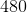dollars, how much money do you owe them?  Round your answer to the nearest cent.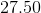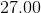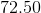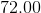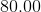Explanation:

To solve this problem, first, you must convert the percentage above into a decimal.  To find the decimal equivalent of a percentage, simply move the decimal (if there is none, remember that there is always an implied decimal after the one's digit) to the left twice.  Then, you take your result and multiply it by the initial amount for your answer:### Example Question #35 : How To Find Decimal Equivalent To A Percentage

If 37% of a pie was consumed, what is the decimal equivalence to how much of the pie is missing?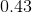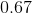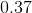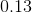Explanation:

This problem is simply asking us to convert 37% into its decimal equivalence.

The quickest way to switch between decimals and percentage is by keeping in mind that it is a factor of 100. For instance, decimals may be converted to percents by multiplying by 100 whereas turning percents to decimals would be the opposite operation (division). This is true because by definition, a percentage is a portion of 100. In this case, we are interested in a portion of 37.

Given this information,

we may convert from percent to decimal. This would require dividing by 100.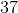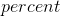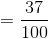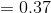### Example Question #36 : How To Find Decimal Equivalent To A Percentage

If Sally cuts 50% of an apple for a pie, what is the decimal equivalence for what has been put into the pie?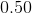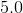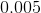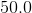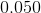Explanation:

This problem is simply asking us to convert 50% into its decimal equivalence.

The quickest way to switch between decimals and percentage is by keeping in mind that it is a factor of 100. For instance, decimals may be converted to percents by multiplying by 100 whereas turning percents to decimals would be the opposite operation (division). This is true because by definition, a percentage is a portion of 100. In this case, we are interested in a portion of 50.

Given this information,

we may convert from percent to decimal. This would require dividing by 100.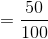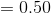### Example Question #37 : How To Find Decimal Equivalent To A Percentage

What is the decimal equivalence of 46%?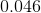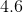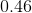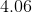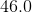Explanation:

This problem is simply asking us to convert 46% into its decimal equivalence.

The quickest way to switch between decimals and percentage is by keeping in mind that it is a factor of 100. For instance, decimals may be converted to percents by multiplying by 100 whereas turning percents to decimals would be the opposite operation (division). This is true because by definition, a percentage is a portion of 100. In this case, we are interested in a portion of 46.

Given this information,

we may convert from percent to decimal. This would require dividing by 100.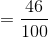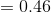### Example Question #38 : How To Find Decimal Equivalent To A Percentage

Find the decimal equivalent of the percent value of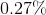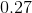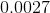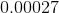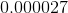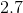Explanation:

To get a decimal value of a percent, we must divide the percent value by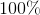: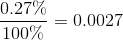### Example Question #39 : How To Find Decimal Equivalent To A Percentage

Which of the following is the decimal equivalent of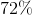?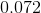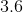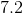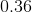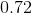Explanation:

To find the decimal equivalent of a percentage, simply move the decimal (if there is none, remember that there is always an implied decimal after the one's digit) to the left twice.

In other words divide the percentage by one hundred.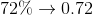or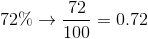### Example Question #40 : How To Find Decimal Equivalent To A Percentage

Which of the following is the decimal equivalent of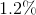?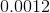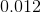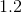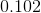Explanation:

To find the decimal equivalent of a percentage, simply move the decimal (if there is none, remember that there is always an implied decimal after the one's digit) to the left twice. In other words, divide the percentage by one hundred.